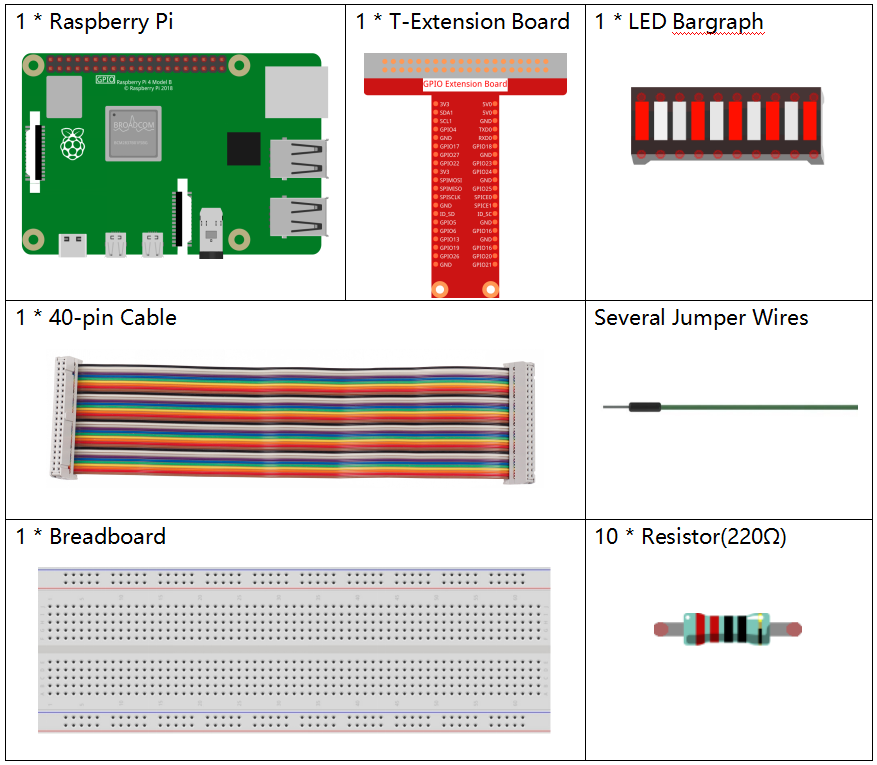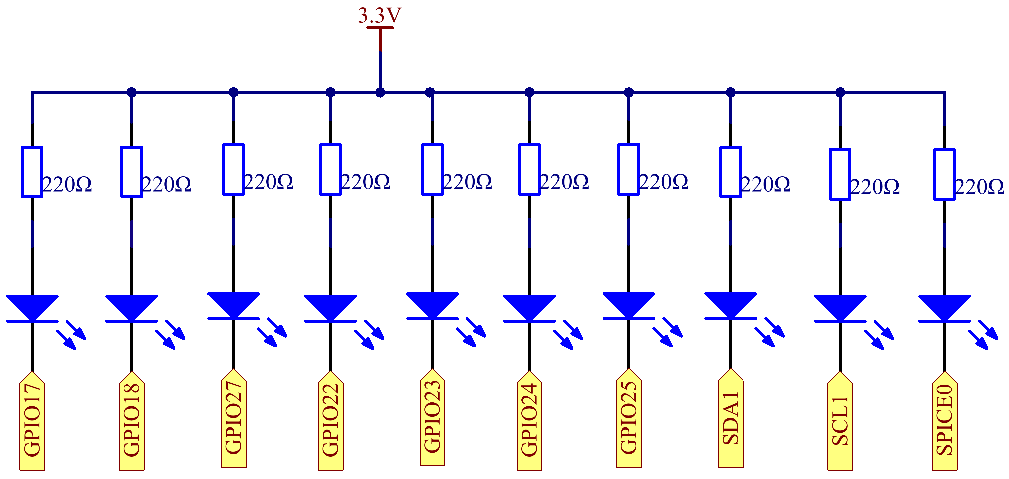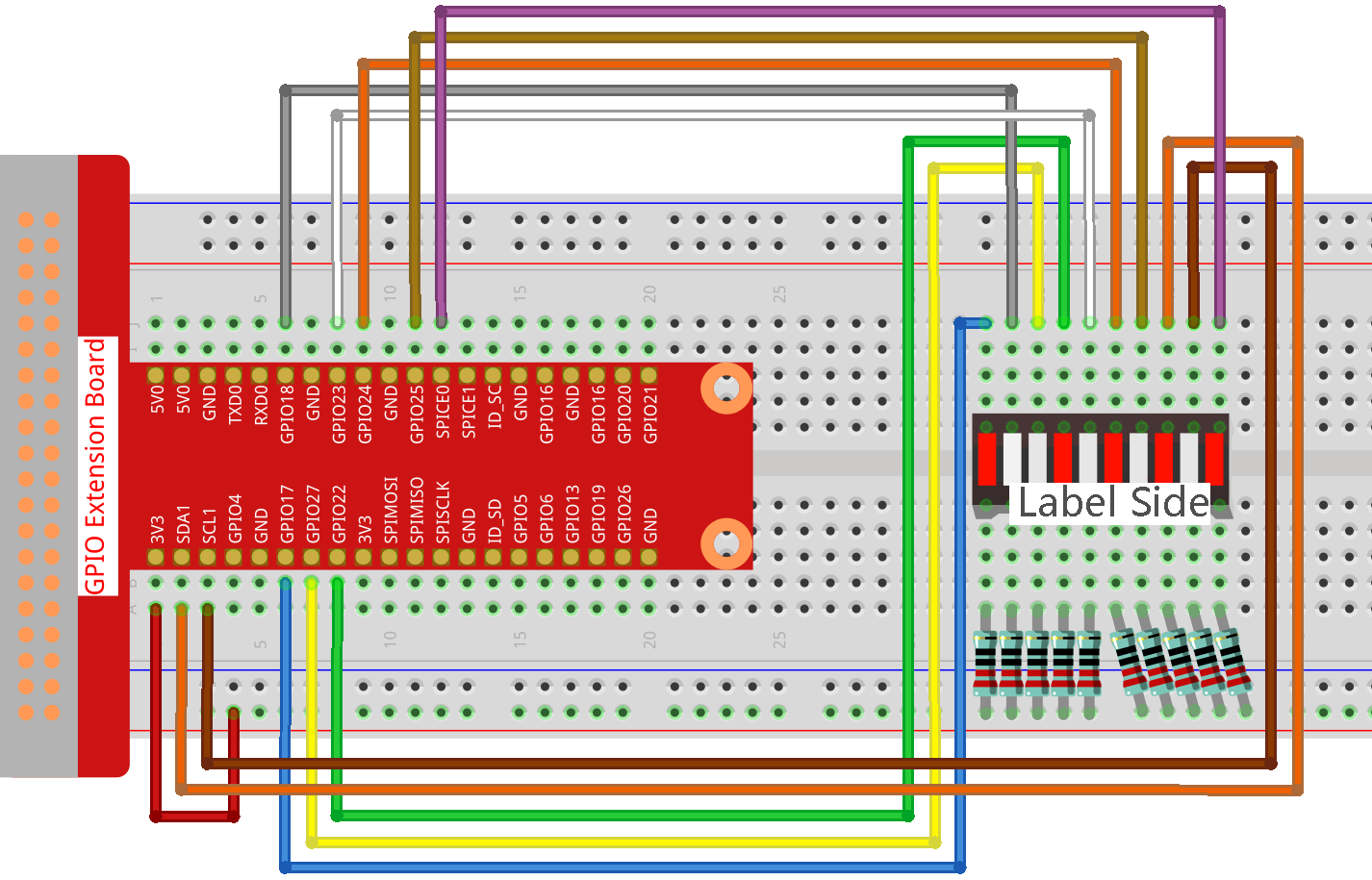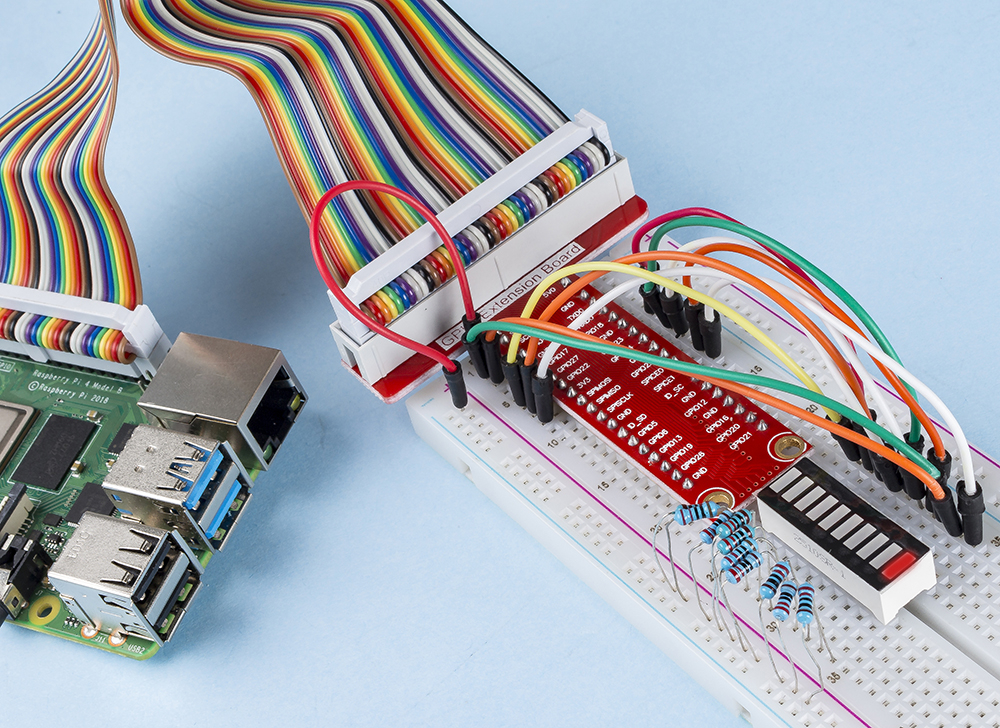# 1.1.3 LED Bar Graph¶

## Introduction¶

In this project, we sequentially illuminate the lights on the LED Bar Graph.

## Required Components¶

In this project, we need the following components.Name

ITEMS IN THIS KIT

Raphael Kit

337

Raphael Kit

COMPONENT INTRODUCTION

GPIO Extension Board

Jumper Wires

Resistor

LED Bar Graph

-

## Schematic Diagram¶

 T-Board Name physical wiringPi BCM GPIO17 Pin 11 0 17 GPIO18 Pin 12 1 18 GPIO27 Pin 13 2 27 GPIO22 Pin 15 3 22 GPIO23 Pin 16 4 23 GPIO24 Pin 18 5 24 GPIO25 Pin 22 6 25 SDA1 Pin 3 8 2 SCL1 Pin 5 9 3 SPICE0 Pin 24 10 8## Experimental Procedures¶

Step 1: Build the circuit.

Note

Pay attention to the direction when connecting. If you connect it backwards, it will not light up.Step 2: Go to the folder of the code.

```cd ~/raphael-kit/python/
```

Step 3: Run the executable file.

```sudo python3 1.1.3_LedBarGraph.py
```

After the code runs, you will see the LEDs on the LED bar turn on and off regularly.

Code

Note

You can Modify/Reset/Copy/Run/Stop the code below. But before that, you need to go to source code path like `raphael-kit/python`. After modifying the code, you can run it directly to see the effect.

```import RPi.GPIO as GPIO
import time

ledPins = [11, 12, 13, 15, 16, 18, 22, 3, 5, 24]

def oddLedBarGraph():
for i in range(5):
j = i*2
GPIO.output(ledPins[j],GPIO.HIGH)
time.sleep(0.3)
GPIO.output(ledPins[j],GPIO.LOW)

def evenLedBarGraph():
for i in range(5):
j = i*2+1
GPIO.output(ledPins[j],GPIO.HIGH)
time.sleep(0.3)
GPIO.output(ledPins[j],GPIO.LOW)

def allLedBarGraph():
for i in ledPins:
GPIO.output(i,GPIO.HIGH)
time.sleep(0.3)
GPIO.output(i,GPIO.LOW)

def setup():
GPIO.setwarnings(False)
GPIO.setmode(GPIO.BOARD)        # Numbers GPIOs by physical location
for i in ledPins:
GPIO.setup(i, GPIO.OUT)   # Set all ledPins' mode is output
GPIO.output(i, GPIO.LOW) # Set all ledPins to high(+3.3V) to off led

def loop():
while True:
oddLedBarGraph()
time.sleep(0.3)
evenLedBarGraph()
time.sleep(0.3)
allLedBarGraph()
time.sleep(0.3)

def destroy():
for pin in ledPins:
GPIO.output(pin, GPIO.LOW)    # turn off all leds
GPIO.cleanup()                     # Release resource

if __name__ == '__main__':     # Program start from here
setup()
try:
loop()
except KeyboardInterrupt:  # When 'Ctrl+C' is pressed, the program destroy() will be  executed.
destroy()
```

Code Explanation

ledPins = [11, 12, 13, 15, 16, 18, 22, 3, 5, 24] Create an array and assign it to the pin number corresponding to the LED Bar Graph (11, 12, 13, 15, 16, 18, 22, 3, 5, 24) and the array will be used to control the LED.

```def oddLedBarGraph():
for i in range(5):
j = i*2
GPIO.output(ledPins[j],GPIO.HIGH)
time.sleep(0.3)
GPIO.output(ledPins[j],GPIO.LOW)
```

Let the LED on the odd digit of the LED Bar Graph light on in turn.

```def evenLedBarGraph():
for i in range(5):
j = i*2+1
GPIO.output(ledPins[j],GPIO.HIGH)
time.sleep(0.3)
GPIO.output(ledPins[j],GPIO.LOW)
```

Make the LED on the even digit of the LED Bar Graph light on in turn.

```def allLedBarGraph():
for i in ledPins:
GPIO.output(i,GPIO.HIGH)
time.sleep(0.3)
GPIO.output(i,GPIO.LOW)
```

Let the LED on the LED Bar Graph light on one by one.

Phenomenon Picture